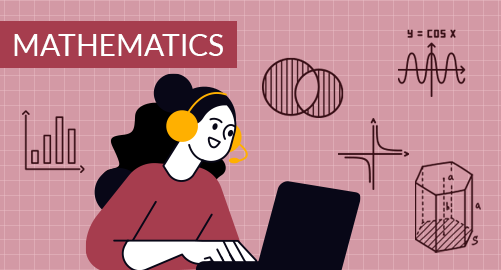#### Need Help?

Get in touch with usMathematics

Grade 6 Mathematics cover Use Positive rational numbers, Integers and rational numbers, Numeric and algebraic expressions, Understand and use ratio, rate and percent, Solve Area, Surface area, and Volume problems, Display, describe, and summarize data

## What you'll learn

• 1

Use Positive rational numbers: Fluently add, subtract, and multiply decimals, Fluently divide whole numbers and decimals, Multiply fractions, Understand division with fractions, Divide fractions by fractions, Divide mixed numbers, Solve problems with rational numbers.

• 2

Integers and rational numbers: Understand Integers, Represent rational numbers on the number line, Absolute values of rational numbers, Represent rational numbers on the coordinate plane, Find distances on the coordinate plane, Represent polygons on the coordinate plane.

• 3

Numeric and algebraic expressions: Understand and represent exponents, Find greatest common factor and least common multiple, Write and evaluate numerical expressions, Write algebraic expressions, Evaluate algebraic expressions, Generate equivalent expressions, Simplify algebraic expressions.

• 4

Represent and solve equations and inequalities: Understand equations and solutions, Apply properties of equality, Write and solve addition, subtraction, multiplication and division equations, Write and solve equations with rational numbers and decimals, Solve inequalities, Understand dependent and independent variables, Use patterns to write and solve equations, Relate tables, graphs, and equations.

• 5

Understand and use ratio, rate and percent: Understand ratios, Generate equivalent ratios, Compare ratios&Represent and graph ratios, Compare, solve unit rates, Convert customary units and metric units, Understand percent, Relate fractions, decimals, and percents, Find the percent of a number, Find the whole given a part and the percent.

• 6

Solve Area, Surface area, and Volume problems: Find areas of parallelogram and rhombuses, Solve triangle area problems, Find areas of trapezoids and polygons, Represent solid figures using nets, Find surface areas of prisms and pyramids, Find volume with fractional edge lengths.

• 7

Display, describe, and summarize data: Recognize statistical questions, Summarize data using mean, median, mode and range, Display data in box plots and frequency tables and histograms, Summarize data using measures of variability, Choose appropriate statistical measures, Summarize data distributions.

## Skills you'll learnIdentify an appropriate mathematical rule or procedure based on the classification of a given expressionExplain how an approximated value relates to the actual valueIdentify mathematical information from graphical, numerical, analytical, and/or verbal representationsDescribe the relationships among different representations of functions and their derivativesExplain the meaning of mathematical solutions in contextIdentify an appropriate mathematical definition, theorem, or test to applyUse precise mathematical language and appropriate units of measure and symbols